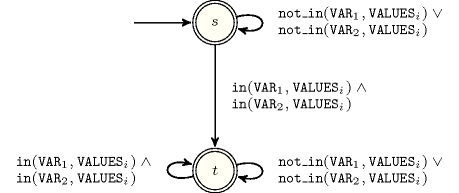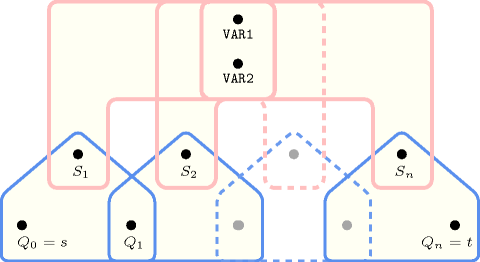## 5.182. in_same_partition

Origin

Used for defining several entries of this catalog.

Constraint

$\mathrm{𝚒𝚗}_\mathrm{𝚜𝚊𝚖𝚎}_\mathrm{𝚙𝚊𝚛𝚝𝚒𝚝𝚒𝚘𝚗}\left(\mathrm{𝚅𝙰𝚁}\mathtt{1},\mathrm{𝚅𝙰𝚁}\mathtt{2},\mathrm{𝙿𝙰𝚁𝚃𝙸𝚃𝙸𝙾𝙽𝚂}\right)$

Type
 $\mathrm{𝚅𝙰𝙻𝚄𝙴𝚂}$ $\mathrm{𝚌𝚘𝚕𝚕𝚎𝚌𝚝𝚒𝚘𝚗}\left(\mathrm{𝚟𝚊𝚕}-\mathrm{𝚒𝚗𝚝}\right)$
Arguments
 $\mathrm{𝚅𝙰𝚁}\mathtt{1}$ $\mathrm{𝚍𝚟𝚊𝚛}$ $\mathrm{𝚅𝙰𝚁}\mathtt{2}$ $\mathrm{𝚍𝚟𝚊𝚛}$ $\mathrm{𝙿𝙰𝚁𝚃𝙸𝚃𝙸𝙾𝙽𝚂}$ $\mathrm{𝚌𝚘𝚕𝚕𝚎𝚌𝚝𝚒𝚘𝚗}\left(𝚙-\mathrm{𝚅𝙰𝙻𝚄𝙴𝚂}\right)$
Restrictions
 $|\mathrm{𝚅𝙰𝙻𝚄𝙴𝚂}|\ge 1$ $\mathrm{𝚛𝚎𝚚𝚞𝚒𝚛𝚎𝚍}$$\left(\mathrm{𝚅𝙰𝙻𝚄𝙴𝚂},\mathrm{𝚟𝚊𝚕}\right)$ $\mathrm{𝚍𝚒𝚜𝚝𝚒𝚗𝚌𝚝}$$\left(\mathrm{𝚅𝙰𝙻𝚄𝙴𝚂},\mathrm{𝚟𝚊𝚕}\right)$ $\mathrm{𝚛𝚎𝚚𝚞𝚒𝚛𝚎𝚍}$$\left(\mathrm{𝙿𝙰𝚁𝚃𝙸𝚃𝙸𝙾𝙽𝚂},𝚙\right)$ $|\mathrm{𝙿𝙰𝚁𝚃𝙸𝚃𝙸𝙾𝙽𝚂}|\ge 2$
Purpose

Enforce $\mathrm{𝚅𝙰𝚁}\mathtt{1}$ and $\mathrm{𝚅𝙰𝚁}\mathtt{2}$ to be respectively assigned to values ${v}_{1}$ and ${v}_{2}$ that both belong to a same partition of the collection $\mathrm{𝙿𝙰𝚁𝚃𝙸𝚃𝙸𝙾𝙽𝚂}$.

Example
$\left(6,2,〈𝚙-〈1,3〉,𝚙-〈4〉,𝚙-〈2,6〉〉\right)$

The $\mathrm{𝚒𝚗}_\mathrm{𝚜𝚊𝚖𝚎}_\mathrm{𝚙𝚊𝚛𝚝𝚒𝚝𝚒𝚘𝚗}$ constraint holds since its first and second arguments $\mathrm{𝚅𝙰𝚁}\mathtt{1}=6$ and $\mathrm{𝚅𝙰𝚁}\mathtt{2}=2$ both belong to the third partition $〈2,6〉$ of its third argument $\mathrm{𝙿𝙰𝚁𝚃𝙸𝚃𝙸𝙾𝙽𝚂}$.

Typical
$\mathrm{𝚅𝙰𝚁}\mathtt{1}\ne \mathrm{𝚅𝙰𝚁}\mathtt{2}$
Symmetries
• Arguments are permutable w.r.t. permutation $\left(\mathrm{𝚅𝙰𝚁}\mathtt{1},\mathrm{𝚅𝙰𝚁}\mathtt{2}\right)$ $\left(\mathrm{𝙿𝙰𝚁𝚃𝙸𝚃𝙸𝙾𝙽𝚂}\right)$.

• Items of $\mathrm{𝙿𝙰𝚁𝚃𝙸𝚃𝙸𝙾𝙽𝚂}$ are permutable.

• Items of $\mathrm{𝙿𝙰𝚁𝚃𝙸𝚃𝙸𝙾𝙽𝚂}.𝚙$ are permutable.

Arg. properties

Extensible wrt. $\mathrm{𝙿𝙰𝚁𝚃𝙸𝚃𝙸𝙾𝙽𝚂}$.

Used in
Keywords
Derived Collection
$\mathrm{𝚌𝚘𝚕}\left(\begin{array}{c}\mathrm{𝚅𝙰𝚁𝙸𝙰𝙱𝙻𝙴𝚂}-\mathrm{𝚌𝚘𝚕𝚕𝚎𝚌𝚝𝚒𝚘𝚗}\left(\mathrm{𝚟𝚊𝚛}-\mathrm{𝚍𝚟𝚊𝚛}\right),\hfill \\ \mathrm{𝚒𝚝𝚎𝚖}\left(\mathrm{𝚟𝚊𝚛}-\mathrm{𝚅𝙰𝚁}\mathtt{1}\right),\mathrm{𝚒𝚝𝚎𝚖}\left(\mathrm{𝚟𝚊𝚛}-\mathrm{𝚅𝙰𝚁}\mathtt{2}\right)\right]\hfill \end{array}\right)$
Arc input(s)

$\mathrm{𝚅𝙰𝚁𝙸𝙰𝙱𝙻𝙴𝚂}$ $\mathrm{𝙿𝙰𝚁𝚃𝙸𝚃𝙸𝙾𝙽𝚂}$

Arc generator
$\mathrm{𝑃𝑅𝑂𝐷𝑈𝐶𝑇}$$↦\mathrm{𝚌𝚘𝚕𝚕𝚎𝚌𝚝𝚒𝚘𝚗}\left(\mathrm{𝚟𝚊𝚛𝚒𝚊𝚋𝚕𝚎𝚜},\mathrm{𝚙𝚊𝚛𝚝𝚒𝚝𝚒𝚘𝚗𝚜}\right)$

Arc arity
Arc constraint(s)
$\mathrm{𝚒𝚗}$$\left(\mathrm{𝚟𝚊𝚛𝚒𝚊𝚋𝚕𝚎𝚜}.\mathrm{𝚟𝚊𝚛},\mathrm{𝚙𝚊𝚛𝚝𝚒𝚝𝚒𝚘𝚗𝚜}.𝚙\right)$
Graph property(ies)
 $•$$\mathrm{𝐍𝐒𝐎𝐔𝐑𝐂𝐄}$$=2$ $•$$\mathrm{𝐍𝐒𝐈𝐍𝐊}$$=1$

Graph model

$\mathrm{𝚅𝙰𝚁}\mathtt{1}$ and $\mathrm{𝚅𝙰𝚁}\mathtt{2}$ are put together in the derived collection $\mathrm{𝚅𝙰𝚁𝙸𝙰𝙱𝙻𝙴𝚂}$. Since both $\mathrm{𝚅𝙰𝚁}\mathtt{1}$ and $\mathrm{𝚅𝙰𝚁}\mathtt{2}$ should take their value in one of the partition depicted by the $\mathrm{𝙿𝙰𝚁𝚃𝙸𝚃𝙸𝙾𝙽𝚂}$ collection, the final graph should have two sources corresponding respectively to $\mathrm{𝚅𝙰𝚁}\mathtt{1}$ and $\mathrm{𝚅𝙰𝚁}\mathtt{2}$. Since two, possibly distinct, values should be assigned to $\mathrm{𝚅𝙰𝚁}\mathtt{1}$ and $\mathrm{𝚅𝙰𝚁}\mathtt{2}$ and since these values belong to the same partition $p$ the final graph should only have one sink. This sink corresponds in fact to partition $p$.

Parts (A) and (B) of Figure 5.182.1 respectively show the initial and final graph associated with the Example slot. Since we both use the $\mathrm{𝐍𝐒𝐎𝐔𝐑𝐂𝐄}$ and $\mathrm{𝐍𝐒𝐈𝐍𝐊}$ graph properties, the source and sink vertices of the final graph are shown with a double circle.

##### Figure 5.182.1. Initial and final graph of the $\mathrm{𝚒𝚗}_\mathrm{𝚜𝚊𝚖𝚎}_\mathrm{𝚙𝚊𝚛𝚝𝚒𝚝𝚒𝚘𝚗}$ constraint(a) (b)
Signature

Note that the sinks of the initial graph cannot become sources of the final graph since isolated vertices are eliminated from the final graph. Since the final graph contains two sources it also includes one arc between a source and a sink. Therefore the minimum number of sinks of the final graph is equal to one. So we can rewrite $\mathrm{𝐍𝐒𝐈𝐍𝐊}=1$ to $\mathrm{𝐍𝐒𝐈𝐍𝐊}\ge 1$ and simplify $\underline{\overline{\mathrm{𝐍𝐒𝐈𝐍𝐊}}}$ to $\overline{\mathrm{𝐍𝐒𝐈𝐍𝐊}}$.

Automaton

Figure 5.182.2 depicts the automaton associated with the $\mathrm{𝚒𝚗}_\mathrm{𝚜𝚊𝚖𝚎}_\mathrm{𝚙𝚊𝚛𝚝𝚒𝚝𝚒𝚘𝚗}$ constraint. Let ${\mathrm{𝚅𝙰𝙻𝚄𝙴𝚂}}_{i}$ be the $𝚙$ attribute of the ${i}^{th}$ item of the $\mathrm{𝙿𝙰𝚁𝚃𝙸𝚃𝙸𝙾𝙽𝚂}$ collection. To each triple $\left(\mathrm{𝚅𝙰𝚁}\mathtt{1},\mathrm{𝚅𝙰𝚁}\mathtt{2},{\mathrm{𝚅𝙰𝙻𝚄𝙴𝚂}}_{i}\right)$ corresponds a 0-1 signature variable ${S}_{i}$ as well as the following signature constraint: $\left(\left(\mathrm{𝚅𝙰𝚁}\mathtt{1}\in {\mathrm{𝚅𝙰𝙻𝚄𝙴𝚂}}_{i}\right)\wedge \left(\mathrm{𝚅𝙰𝚁}\mathtt{2}\in {\mathrm{𝚅𝙰𝙻𝚄𝙴𝚂}}_{i}\right)\right)⇔{S}_{i}$.

##### Figure 5.182.2. Automaton of the $\mathrm{𝚒𝚗}_\mathrm{𝚜𝚊𝚖𝚎}_\mathrm{𝚙𝚊𝚛𝚝𝚒𝚝𝚒𝚘𝚗}$ constraint##### Figure 5.182.3. Hypergraph of the reformulation corresponding to the automaton of the $\mathrm{𝚒𝚗}_\mathrm{𝚜𝚊𝚖𝚎}_\mathrm{𝚙𝚊𝚛𝚝𝚒𝚝𝚒𝚘𝚗}$ constraint